# AP Physics B : Understanding Vector and Scalar Quantities

## Example Questions

### Example Question #1 : Understanding Vector And Scalar Quantities

A ball is thrown at a velocity of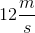at an angle of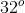from the horizontal. What are the ball's horizontal and vertical velocities?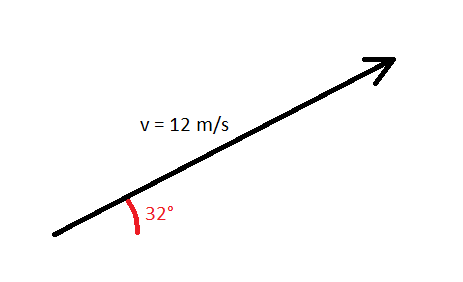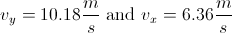There is not enough information to solve this problem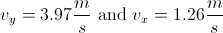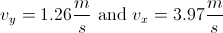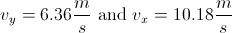Explanation:

The velocity ofcan be broken into horizontal and vertical components by using trigonometry. Think of the figure below, where x and y velocity components of the total velocity are shown.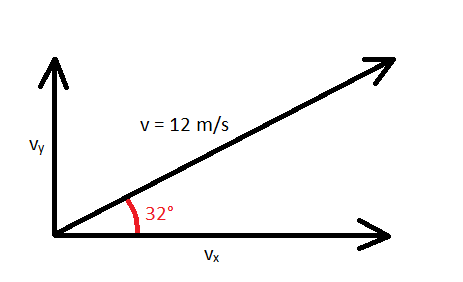Use the total velocity, the x-component, and the y-component to form a right triangle below.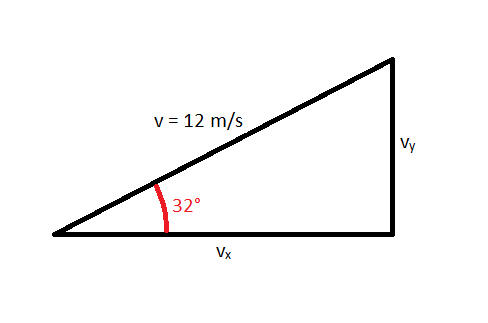Treatingas the hypotenuse, x-component as the leg adjacent, and y-component as the leg opposite, you can conclude that the velocities are related through trigonometric identities.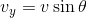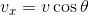Plugging in the given values, we can solve for the x and y velocity components.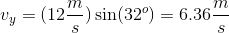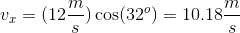### Example Question #1 : Newtonian Mechanics

Which answer choice below includes only scalar quantities?

Force, time, velocity

Acceleration, energy, displacement

Displacement, time, acceleration

Velocity, displacement, force

Distance, speed, time